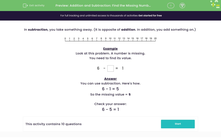# Find the Missing Number

In this worksheet, students will solve missing number problems using subtraction (0-20).Key stage:  KS 1

Curriculum topic:   Number: Addition and Subtraction

Curriculum subtopic:   Solve Add/Subtract Problems to 20

Popular topics:   Subtraction worksheets, Addition worksheets

Difficulty level:#### Worksheet Overview

In this activity, we will be working out the missing number in a number sentence.

We can work out the answer using addition or subtraction -  it just depends on which number in the number sentence is missing.

Sometimes the missing number will be the second number in the number sentence:

 6 - = 1

Sometimes it will be the first number:

 - 4 = 1

Let's look at how we find the missing number when it is the second number in the number sentence.

Example 1

 6 - = 1

The numbers in a number sentence all link up, we just need to move them around. Here, we can subtract the 1 from 6 to get the missing number.

6 - 1 = 5

Now, we can put the 5 back into the original number sentence and check it works.

 6 - 5 = 1

Let's check if this has worked. Put your finger on 6 and jump back 5. We have landed on the number 1 - this means 6 - 5 = 1 so it has worked!So, when the second number is missing in a number sentence, we can find out what the answer is by just subtracting the other number from the first number.

Example 2

Look at this problem. This time, the first number in the number sentence is missing. We work this one out a different way!

 - 7 = 2

As it is the first number that is missing, the first number in a subtraction sentence is always the biggest. This means that we need to use addition to work it out. We take the number we have and add them together to find the missing number.

7 + 2 = 9

Now we need to check it has worked! Let's put 9 into the missing space:

 9 - 7 = 2

Let's check if this has worked, put your finger on 9 and jump back 7. We have landed on the number 2 - this means 9 - 7 = 2 so it has worked!Let's try some questions now and see how we get on.

### What is EdPlace?

We're your National Curriculum aligned online education content provider helping each child succeed in English, maths and science from year 1 to GCSE. With an EdPlace account you’ll be able to track and measure progress, helping each child achieve their best. We build confidence and attainment by personalising each child’s learning at a level that suits them.

Get started